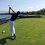# The Game

There are two players, player $A$ with data set $A$ and elements $a_i$ and player $B$ with data set $B$ and elements $b_i$. The arithmetic mean of each data set is 10 and 20, respectively.

A "game" is played in which each $a_i$ is compared to its corresponding $b_i$ with the same base. If the element from set $A$ is less than its corresponding element from set $B$, then player $A$ is awarded 1 point and visa versa with player $B$.

What is the probability that player $B$ has more points than player $A$

You may manipulate the following or exclude them if they are not necessary. This is to either a) make this problem easier, or b) to make the problem possible.

A) The range of the data in both sets. (Make this $0,\infty$ if possible)

B) The number of elements in each set. Notes:

$~~~~~$ • The number of elements in sets $A$ and $B$ must be the same

$~~~~~$ • The number of elements in set $A$ determines the number of matches.

$~~~~~$ • I don't think the number of elements matters, but it mightNote by Trevor Arashiro
6 years, 2 months ago

This discussion board is a place to discuss our Daily Challenges and the math and science related to those challenges. Explanations are more than just a solution — they should explain the steps and thinking strategies that you used to obtain the solution. Comments should further the discussion of math and science.

When posting on Brilliant:

• Use the emojis to react to an explanation, whether you're congratulating a job well done , or just really confused .
• Ask specific questions about the challenge or the steps in somebody's explanation. Well-posed questions can add a lot to the discussion, but posting "I don't understand!" doesn't help anyone.
• Try to contribute something new to the discussion, whether it is an extension, generalization or other idea related to the challenge.
• Stay on topic — we're all here to learn more about math and science, not to hear about your favorite get-rich-quick scheme or current world events.

MarkdownAppears as
*italics* or _italics_ italics
**bold** or __bold__ bold
- bulleted- list
• bulleted
• list
1. numbered2. list
1. numbered
2. list
Note: you must add a full line of space before and after lists for them to show up correctly
paragraph 1paragraph 2

paragraph 1

paragraph 2

[example link](https://brilliant.org)example link
> This is a quote
This is a quote
    # I indented these lines
# 4 spaces, and now they show
# up as a code block.

print "hello world"
# I indented these lines
# 4 spaces, and now they show
# up as a code block.

print "hello world"
MathAppears as
Remember to wrap math in $$ ... $$ or $ ... $ to ensure proper formatting.
2 \times 3 $2 \times 3$
2^{34} $2^{34}$
a_{i-1} $a_{i-1}$
\frac{2}{3} $\frac{2}{3}$
\sqrt{2} $\sqrt{2}$
\sum_{i=1}^3 $\sum_{i=1}^3$
\sin \theta $\sin \theta$
\boxed{123} $\boxed{123}$

## Comments

Sort by:

Top Newest

So many possibilities here, depending on the particular distributions. A "game theory" question could go like this: Suppose player $A$ must choose $N$ (positive integral) scores with the only stipulation that they have a mean of $\mu_{A}$, and player $B$ must similarly choose $N$ (positive integral) scores that have a mean of $\mu_{B}.$ The scores are then ordered by each players and compared, in order, to those of the other player with the tally system you have indicated. What is the best strategy for the two players to achieve the maximum respective tally? Given that the two play optimally, what is the likely final tally as a function of $N, \mu_{A}$ and $\mu_{B}$? I know that this isn't quite what you had in mind, but I thought I'd just throw it out there, anyway. :)

- 6 years, 2 months ago

Log in to reply

Yes, this is a much more feasible task. That turns this from more of a computer science problem into a combinatorics/NT problem.

- 6 years, 2 months ago

Log in to reply

Assuming that the number of elements in each set is the same and knowing the fact that the arithmetic mean from B is two times as the arithmetic mean from A, I think the probabilitiy is 1/2

- 5 years, 11 months ago

Log in to reply

×

Problem Loading...

Note Loading...

Set Loading...